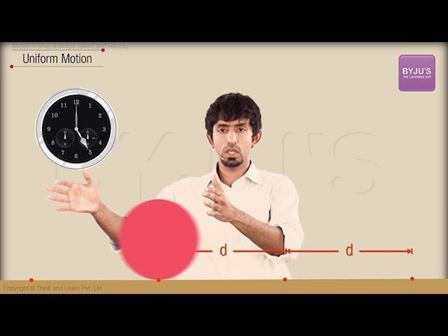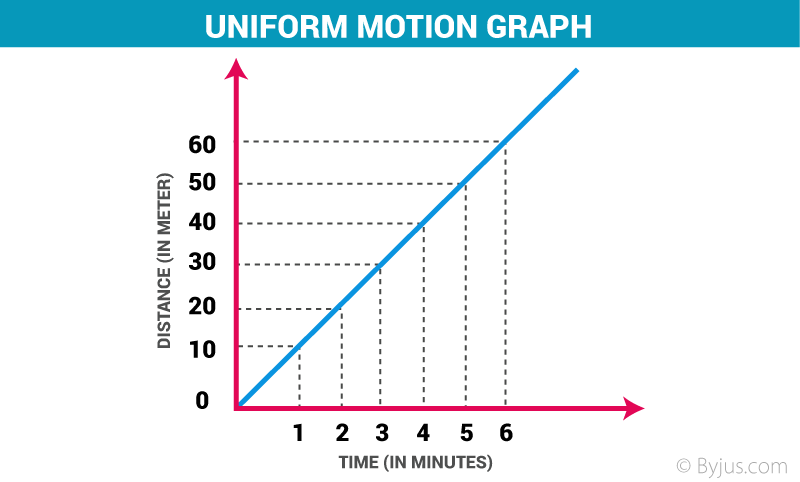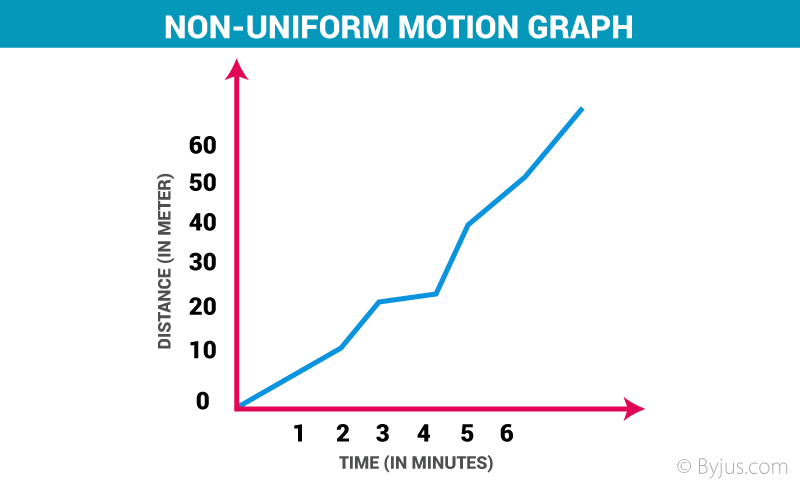# Uniform Motion and Non Uniform Motion## Uniform Motion:

Definition: This type of motion is defined as the motion of an object in which the object travels in a straight line and its velocity remains constant along that line as it covers equal distances in equal intervals of time, irrespective of the duration of the time.If a body is involved in rectilinear motion and the motion is consistent, then the acceleration of the body must be zero.

### Example of Uniform Motion:

1. If the speed of a car is 10 m/s, it means that the car covers 10 meters in one second. The speed is constant in every second.
2. Movement of blades of a ceiling fan.

## Non Uniform Motion:

Definition: This type of motion is defined as the motion of an object in which the object travels with varied speed and it does not cover same distance in equal time intervals, irrespective of the time interval duration.If a body is involved in rectilinear motion, and if the motion is not consistent, then the acceleration of the body must be non-zero.

### Example of Non Uniform Motion:

1. If a car covers 10 meters in first two seconds, and 15 meters in next two seconds.
2. The motion of a train.

Now, people usually get confused between uniform motion and uniform acceleration. In the later phenomena, the object is having a constant acceleration in rectilinear motion, which means the object has different speed in every second, which clearly defines that motion is changing.

Following is the table explaining concepts related to motion

### Comparison table Between these two types of motions:

Comparison Parameters

Uniform Motion

Non Uniform Motion

Average Speed

The motion is similar to the actual speed of the object.

The motion is different from the actual speed of the object.

Rectilinear Motion

It has zero acceleration.

It has non-zero acceleration.

Graph

Distance-time graph shows a straight line

Distance-time graph shows a curved line

Distance

Covers equal distances in equal time interval.

Covers unequal distances in equal time interval.

### Uniform Circular Motion:

This type of motion is seen if an object is travelling at a constant speed around a fixed axis or center point, and the object travels around a curved path and it maintains a constant radial distance from the center point at any given time and it moves in a tangent to the curved path.

### Non Uniform Circular Motion:

This type of motion is inconsistent because if an object is travelling at a variable angular speed around a fixed axis or center point, then the motion of that object is said to be inconsistent because the object covers a curved path and it will have some variable radial acceleration due to which its velocity would change every second.

#### 1 Comment

1. Vivek kumar gupta

Thanks